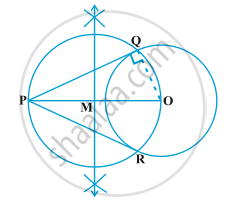# Construction of Tangents to a Circle

#### description

• Construction of Tangent to the Circle from the Point Out Side the Circle
• To construct the tangents to a circle from a point outside it

#### notes

Construction 11.3 : To construct the tangents to a circle from a point outside it.

Steps of Construction:1) Draw a circle with centre O.

2) Take a point P outside circle.

3) Join PO. With the help of compass we bisect PO.

4) Let M be the midpoint of PO.

5) Taking M as centre & MO as radius, draw circle.

6) Let it intersect the given circle at Q and R.

7) Join PQ and PR.

8) PQ and PR are required 2 tangents.

If you would like to contribute notes or other learning material, please submit them using the button below.

### Shaalaa.com

Constructions part 3 (Draw tangent to a circle) [00:02:42]
S
0%